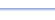Program: VOLUMES

VOLUMES

• Computes the volume of a sphere, cylinder, box (rectangular prism), cone, or pyramid.

Useful for:

• SAT
• ACT
• Algebra 1
• Algebra 2
• Geometry
• Precalculus
• Trigonometry
• Calculus

See full list of programs

Example

Q: Find the volume of a cone with radius 5 and height 8.

Solution:

Run VOLUMES.  How?

Select choice 4
for 'CONE'.

Enter:
R = 5
H = 8

The program reports:

Interpretation: